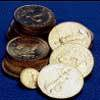#### You may also like### Five Coins

Ben has five coins in his pocket. How much money might he have?### Christmas Shopping

Vera is shopping at a market with these coins in her purse. Which things could she give exactly the right amount for?Lolla bought a balloon at the circus. She gave the clown six coins to pay for it. What could Lolla have paid for the balloon?

# The Money Maze

##### Age 7 to 11Challenge Level

We received several well reasoned solutions to The Money Maze. Lucy and Hayley from Thomas Reade Primary School and Ben from St Michael's C of E Primary School sent particularly clear solutions where they showed each step in turn. Here is Lucy and Hayley's contribution:

The highest integer:
1. $100 (-10)=$
2. $90 (+150)=$
3. $240 (-50)=$
4. $190 (+120)=$
8. $310 (-20)=$
7. $290 (+145(+50$%$))=$
6. $435 (-50)=$
10. $385 (\times2)=$
11. $770 (-10)=$
15. $760 (+30)=$
16. $790$!!!

Lowest integer:
1. $100 (-50)=$
5. $50 (+10)=$
9. $60 (-50)=$
10. $10 (+5(+50$%$))=$
6. $15 (-20)=$
7. $-5 (\times2)=$
11. $-10 (-10)=$
15. $-20 (+30)=$
16. $10$!!!

I like the way you have presented this, girls, it makes it easy to follow the operations.

A teacher from St Luke's Church of England Primary School Westbeams Road, Sway, Hampshire, sent in the following email.

One of my students, Jasper  has this morning completed this challenge and found a different answer to the second part of the problem to the one published.

Before looking at the solution, he came up with the answer as £40, following this route: